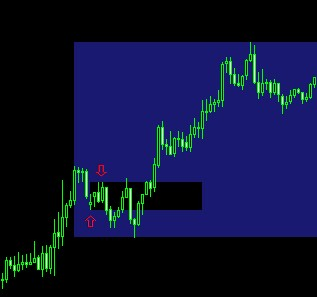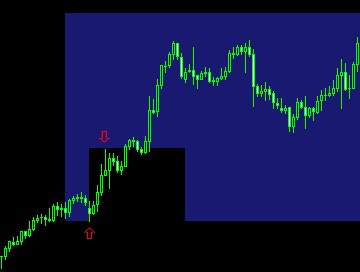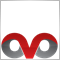# How to find the first swing high and low of a range107

Pretty much as the title states, how can I find the first swing high and swing low of a bigger range?3526

remix919:

Pretty much as the title states, how can I find the first swing high and swing low of a bigger range?

you cannot find a swing, unless you exactly express, what you consider to be a swing.19144

```//+------------------------------------------------------------------+
//| Find a local extreme bar value                                   |
//+------------------------------------------------------------------+
int MaximalBar(int length, int start=0, int TF=0, double d=INF){
if (d>=INF) d=DIR;
if (Bars-start < length)   length = Bars - start;
if (d>0)    return( Highest(NULL, TF, MODE_HIGH, length, start) );
else     return(  Lowest(NULL, TF, MODE_LOW,  length, start) );
}
int LocalExtreme(int WS, int first=0, int TF=0, double d=INF){
while(true){                                             int
firstPrev   = first;
first    = MaximalBar(WS, first, TF, d);
if (first == firstPrev)    return(first);
}
/*NOTREACHED*/
}  // LocalExtreme
double LastFractal(int WS, int TF=0, double d=INF){
for (int shift=1; shift<Bars; shift++){
int LE = LocalExtreme(WS, shift, TF, d);
if (LE >= shift+2)   return (LE);
}
return(Bars-1);
}
```107

Ovo:
you cannot find a swing, unless you exactly express, what you consider to be a swing.
The FIRST swing high and swing low in this case would simply be the first high and low fractal within a range.107

WHRoeder: